# A Brief Introduction To Generative Adversarial Networks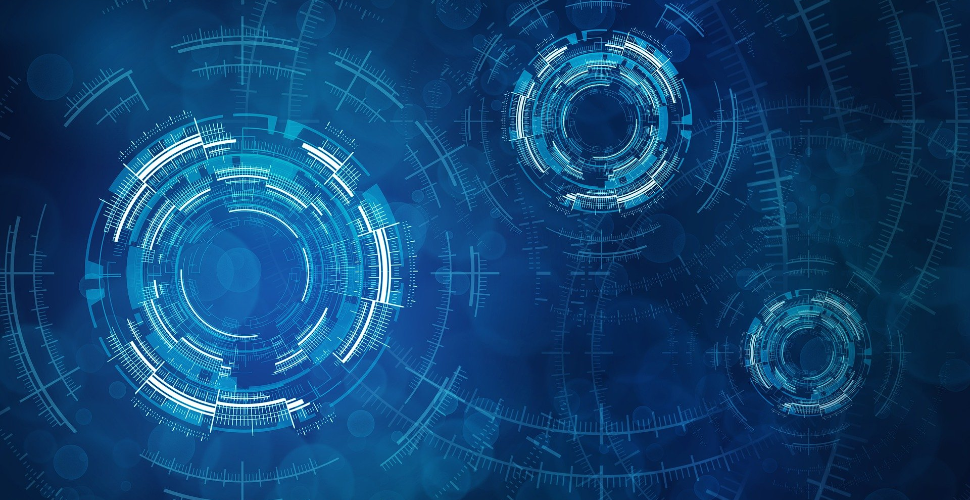# A Brief Introduction To Generative Adversarial Networks

Generative Adversarial Networks (GAN) is one of the most promising recent developments in Deep Learning. GAN, introduced by Ian Goodfellow in 2014, attacks the problem of unsupervised learning by training two deep networks, called Generator and Discriminator, that compete and cooperate with each other. In the course of training, both networks eventually learn how to perform their tasks.

GANs are like the case of a counterfeiter (Generative) and the police (Discriminator). Initially, the counterfeiter will show the police a fake money. The police says it is fake. The police gives feedback to the counterfeiter why the money is fake. The counterfeiter attempts to make a new fake money based on the feedback it received. The police says the money is still fake and offers a new set of feedback. The counterfeiter attempts to make a new fake money based on the latest feedback. The cycle continues indefinitely until the police is fooled by the fake money because it looks real.

While the idea of GAN is simple in theory, it is very difficult to build a model that works. In GAN, there are two deep networks coupled together making back propagation of gradients twice as challenging. Deep Convolutional GAN (DCGAN) is one of the models that demonstrated how to build a practical GAN that is able to learn by itself how to synthesize new images.

In this article, we discuss how a working DCGAN can be built using Keras 2.0 on Tensorflow 1.0 backend in less than 200 lines of code. We will train a DCGAN to learn how to write handwritten digits, the MNIST way.

### MNIST Handwritten Digit Dataset

The MNIST dataset is an acronym that stands for the Modified National Institute of Standards and Technology dataset. It is a dataset of 70,000 small square 28×28 pixel grayscale images of handwritten single digits between 0 and 9. The task is to classify a given image of a handwritten digit into one of 10 classes representing integer values from 0 to 9, inclusively. Keras provides access to the MNIST dataset via the mnist.load_dataset() function. It returns two tuples, one with the input and output elements for the standard training dataset, and another with the input and output elements for the standard test dataset. The example below loads the dataset and summarizes the shape of the loaded dataset.

``````
# load the images into memory
(trainX, trainy), (testX, testy) = load_data()
# summarize the shape of the dataset
print('Train', trainX.shape, trainy.shape)
print('Test', testX.shape, testy.shape)
```
```

Dataset looks something like this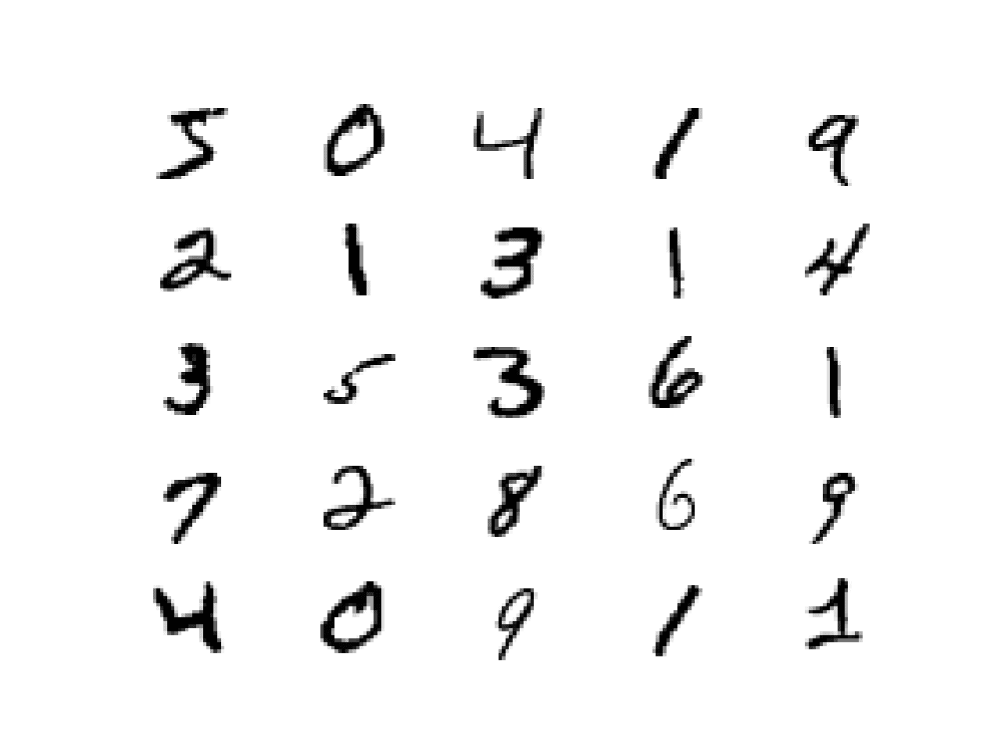Figure 1. MNIST Handwritten Digit Dataset SampleDiscriminator

Job of discriminator is to tells us how real an image is. It is basically a deep Convolutional Neural Network (CNN) as shown in Figure 1. For MNIST Dataset, the input is an image (28 pixel x 28 pixel x 1 channel). The sigmoid output is a scalar value of the probability of how real the image is (0.0 is certainly fake, 1.0 is certainly real, anything in between is a gray area). The difference from a typical CNN is the absence of max-pooling in between layers. Instead, a strided convolution is used for downsampling. The activation function used in each CNN layer is a leaky ReLU. A dropout between 0.4 and 0.7 between layers prevent over fitting and memorization. Listing 1 shows the implementation in Keras.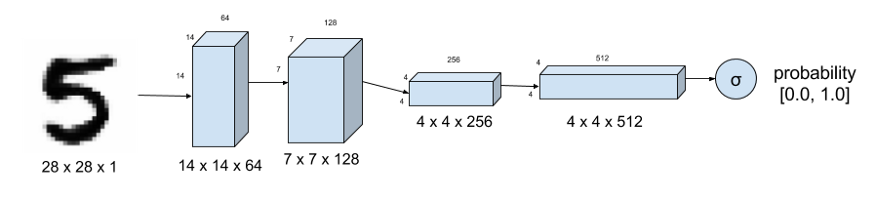Figure 2. DiscriminatorFigure

#### Discriminator

Discriminator of DCGAN tells how real an input image of a digit is. MNIST Dataset is used as ground truth for real images. Strided convolution instead of max-pooling down samples the image.

``````
self.D = Sequential()
depth = 64
dropout = 0.4
# In: 28 x 28 x 1, depth = 1
# Out: 14 x 14 x 1, depth=64
input_shape = (self.img_rows, self.img_cols, self.channel)
# Out: 1-dim probability
self.D.summary()
```
```
Keras code for the Discriminator in Figure 1.

#### Generator

The generator synthesizes fake images. In Figure 2, the fake image is generated from a 100-dimensional noise (uniform distribution between -1.0 to 1.0) using the inverse of convolution, called transposed convolution. Instead of fractionally-strided convolution as suggested in DCGAN, upsampling between the first three layers is used since it synthesizes more realistic handwriting images. In between layers, batch normalization stabilizes learning. The activation function after each layer is a ReLU. The output of the sigmoid at the last layer produces the fake image. Dropout of between 0.3 and 0.5 at the first layer prevents overfitting. Listing 2 shows the implementation in Keras.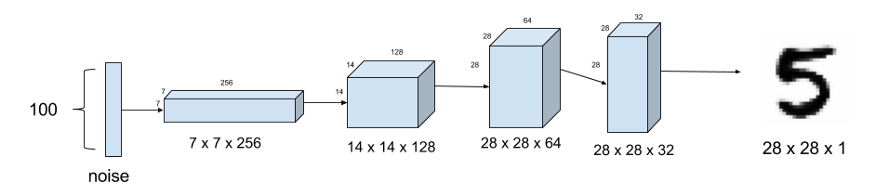Figure 3. GeneratorFigure

Generator model synthesizes fake MNIST images from noise. Upsampling is used instead of fractionally-strided transposed convolution.

``````
self.G = Sequential()
dropout = 0.4
depth = 64+64+64+64
dim = 7
# In: 100
# Out: dim x dim x depth
# In: dim x dim x depth
# Out: 2*dim x 2*dim x depth/2
# Out: 28 x 28 x 1 grayscale image [0.0,1.0] per pix
self.G.summary()
return self.G
```
```

Keras code for the generator in Figure 2.

### GAN Model

So far, there are no models yet. It is time to build the models for training. We need two models: 1) Discriminator Model (the police) and 2) Adversarial Model or Generator-Discriminator (the counterfeiter learning from the police).

#### Discriminator Model

Listing 3 shows the Keras code for the Discriminator Model. It is the Discriminator described above with the loss function defined for training. Since the output of the Discriminator is sigmoid, we use binary cross entropy for the loss. RMSProp as optimizer generates more realistic fake images compared to Adam for this case. Learning rate is 0.0008. Weight decay and clip value stabilize learning during the latter part of the training. You have to adjust the decay if you adjust the learning rate.

``````
optimizer = RMSprop(lr=0.0008, clipvalue=1.0, decay=6e-8)
self.DM = Sequential()
self.DM.compile(loss='binary_crossentropy', optimizer=optimizer,\metrics=['accuracy'])
```
```

Discriminator Model implemented in Keras.

The adversarial model is just the generator-discriminator stacked together as shown in Figure 3. The Generator part is trying to fool the Discriminator and learning from its feedback at the same time. Listing 4 shows the implementation using Keras code. The training parameters are the same as in the Discriminator model except for a reduced learning rate and corresponding weight decay.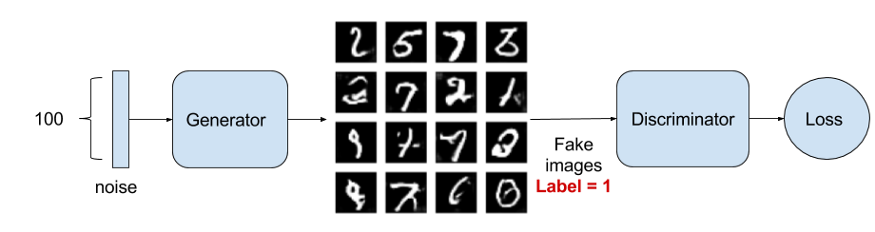The Adversarial model is simply generator with its output connected to the input of the discriminator. Also shown is the training process wherein the Generator labels its fake image output with 1.0 trying to fool the Discriminator.

``````
optimizer = RMSprop(lr=0.0004, clipvalue=1.0, decay=3e-8)
self.AM = Sequential()
self.AM.compile(loss='binary_crossentropy', optimizer=optimizer,\metrics=['accuracy'])
```
```

Adversarial Model as shown in Figure 3 implemented in Keras.

#### Training

Training is the hardest part. We determine first if Discriminator model is correct by training it alone with real and fake images. Afterwards, the Discriminator and Adversarial models are trained one after the other. Figure 4 shows the Discriminator Model while Figure 3 shows the Adversarial Model during training. Listing 5 shows the training code in Keras.Figure 5. Discriminator training

Discriminator model is trained to distinguish real from fake handwritten images.

``````
images_train = self.x_train[np.random.randint(0,self.x_train.shape, size=batch_size), :, :, :]
noise = np.random.uniform(-1.0, 1.0, size=[batch_size, 100])
images_fake = self.generator.predict(noise)
x = np.concatenate((images_train, images_fake))
y = np.ones([2*batch_size, 1])
y[batch_size:, :] = 0
d_loss = self.discriminator.train_on_batch(x, y)
y = np.ones([batch_size, 1])
noise = np.random.uniform(-1.0, 1.0, size=[batch_size, 100])
```
```

Listing 5. Sequential training of Discriminator Model and Adversarial Model. Training steps above 1000 generates respectable outputs.

Training GAN models requires a lot of patience due to its depth. Here are some pointers:

Problem: generated images look like noise.

Solution: use dropout on both Discriminator and Generator. Low dropout values (0.3 to 0.6) generate more realistic images.

Problem: Discriminator loss converges rapidly to zero thus preventing the Generator from learning.

Solution: Do not pre-train the Discriminator. Instead make its learning rate bigger than the Adversarial model learning rate. Use a different training noise sample for the Generator.

Problem: generator images still look like noise.

Solution: check if the activation, batch normalization and dropout are applied in the correct sequence.

Problem: figuring out the correct training/model parameters.

Solution: start with some known working values from published papers and codes and adjust one parameter at a time. Before training for 2000 or more steps, observe the effect of parameter value adjustment at about 500 or 1000 steps.

### Sample Outputs

Figure 5 shows the evolution of output images during training. Observing Figure 5 is fascinating. The GAN is learning how to write handwritten digits on its own!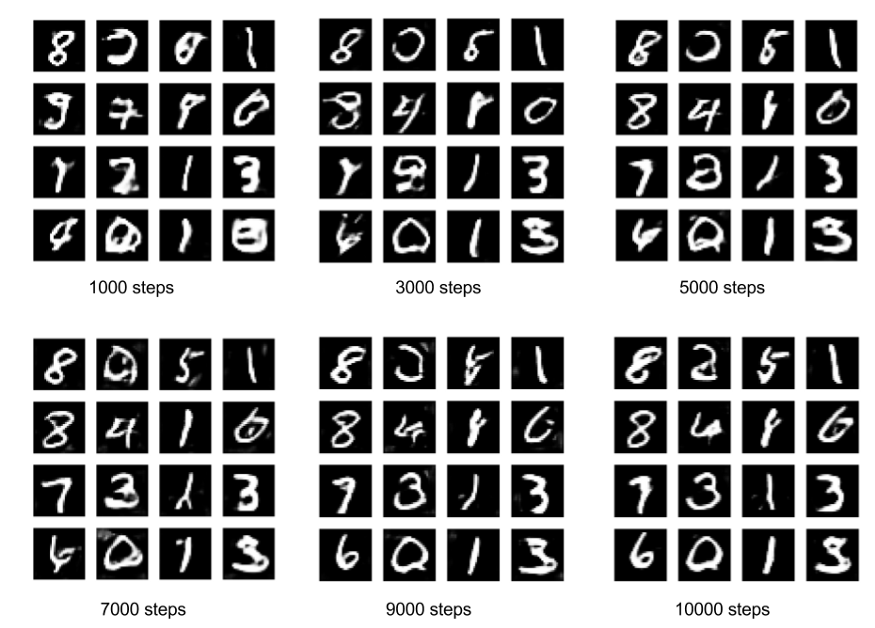Figure 6. DCGAN output images

Figure 6. DCGAN output images during 10,000 steps of training. The Keras complete code can be found here.

#

#

#

#